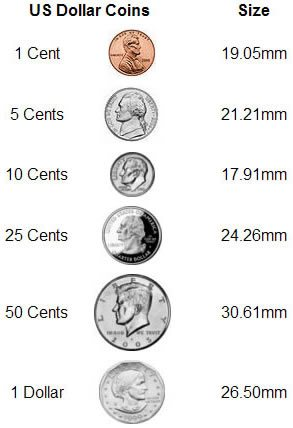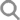サインイン サインアップ カスタマーサポートは英語のみでのご対応となります。 フリーダイアル（アメリカおよびカナダからのお電話のみ）: 1-800-464-1640 国際電話: +66-39303404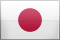USD ここから始める ショッピング ショッピング 新着商品 カボション ペア まとめ買い 標準サイズ ヘルプ ヘルプ わたしたちについて
 Frequently Asked Questions (FAQ) / Specific Gravity & Gemstone Density What is the Meaning of "Specific Gravity" for Gemstones? What Exactly Does it Measure?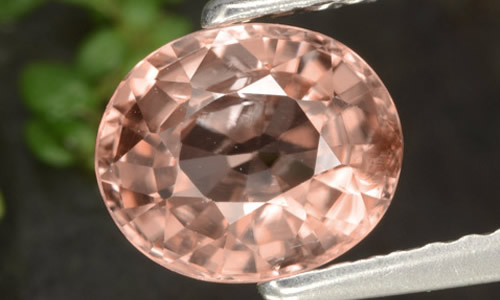Specific gravity is a way to express the relative density of a gemstone. It is measured as the ratio of the density of the gemstone to the density of water, and is expressed as a number that indicates how much heavier the gemstone is compared to an equal volume of water. Scientifically, specific gravity is defined as a ratio of the mass of a given material to the mass of an equal volume of water at 4 degrees centigrade. Most gemstone substances are two to four times denser than an equal volume of water. Specific gravities are expressed in decimal numbers, for example, 4.00 for corundum, 3.52 for diamond and 2.72 for quartz. Zircon is one of the densest gemstones (pictured above); it can have a specific gravity as high as 4.73! To calculate exact specific gravity values, various measuring devices are used. Rough approximations of the specific gravity of lighter stones can be made by using a series of liquids with known specific gravity values. For example, if a stone floats in a liquid with a specific gravity of 4 and sinks in a liquid with a specific gravity of 3, the specific gravity of the stone must lie between these values. See our specific gravity chart for density ratings of the different types of gemstones. Return to FAQ HOME
• 初刊: 2月-20-2006
• 最終アップデート: 9月-12-2017
ウェブサイト上のテキストまたは画像を GemSelect.com (SETT Company Ltd.)の許可なく使用することは固く禁じられています。.
 その他の形状

•  サファイア
•  エメラルド
•  ルビー
•  アクアマリン
•  ジルコン
•  オパール
•  トパーズ
•  トルマリン
•  ガーネット
•  アメジスト
•  シトリン
•  タンザナイト
すべての宝石 (145)
•  アイオライト
•  アイドクレース
•  アクアマリン
•  アクチノライト キャッツアイ
•  アパタイト
•  アベンチュリン
•  アマゾナイト
•  アメジスト
•  アメトリン
•  アルマンディンガーネット
•  アンダルサイト
•  アンデシン ラブラドライト
•  アンモライト
•  インペリアルトパーズ
•  エメラルド
•  エンスタタイト
•  オパール
•  オブシディアン
•  カイヤナイト
•  カラーチェンジガーネット
•  カラーチェンジサファイア
•  カラーチェンジダイアスポア
•  カラーチェンジフローライト
•  カルサイト
•  カルセドニー
•  カーネリアン
•  ガーネット
•  キャッツアイの宝石
•  キャッツアイアクアマリン
•  キャッツアイアパタイト
•  キャッツアイオパール
•  キャッツアイオーガイト
•  キャッツアイスキャポライト
•  キャッツアイムーンストーン
•  クォーツ
•  クォーツ キャッツアイ
•  クリソコラ
•  クリソプレーズ
•  クリソベリル
•  クロムディオプサイド
•  クロムトルマリン
•  クンザイト
•  グランディデライト
•  グロッシュラライト ガーネット
•  コーネルピン
•  ゴールドベリル
•  サファイア
•  サンゴ
•  サンストーン
•  サーペンティン
•  シトリン
•  シリマナイト
•  シリマナイトキャッツアイ
•  ジェダイト
•  ジェード
•  ジャスパー
•  ジルコン
•  スキャポライト
•  スギライト
•  スコレサイト
•  スターガーネット
•  スターサファイア
•  スターサンストーン
•  スターストーン
•  スターディオプサイド
•  スタームーンストーン
•  スタールビー
•  スターレモンクォーツ
•  スターローズクォーツ
•  ストロベチークォーツ
•  スノーフレーク・オブシディアン（黒曜石）
•  スピネル
•  スファレライト
•  スフェーン
•  スペクトロライト
•  スペサルティン ガーネット
•  スミソナイト
•  スモーキークォーツ
•  セラフィナイト
•  ソーダライト
•  タイガーズアイ
•  タイガーズアイマトリックス
•  タンザナイト
•  ターコイズ
•  ダイヤモンド
•  ダブレットオパール
•  チャロアイト
•  チョコレートオパール
•  ツァボライトガーネット
•  デマントイド ガーネット
•  デンドリティックアガート
•  トパーズ
•  トルマリン
•  ドゥルージー アズライト
•  ドルジーアメジスト
•  ヌーマイト
•  ハイアライトオパール
•  ハウライト
•  バリサイト
•  パイライト
•  パイロープガーネット
•  パール
•  ヒデナイト
•  ピーターサイト
•  ファイアーアガート
•  ファイアーオパール
•  フローライト
•  ブラックオパール
•  ブラッドストーン
•  プレナイト
•  ヘソナイトガーネット
•  ヘマタイト
•  ヘミモルファイト ドゥルージー
•  ベリル
•  ペリドット
•  ホークスアイ
•  ボルダーオパール
•  マウ・シット・シット
•  マザーオブパール
•  マトリックスオパール
•  マラカイト
•  マラヤガーネット
•  マリガーネット
•  ミスティッククォーツ
•  ミスティックトパーズ
•  ムーンストーン
•  メノウ
•  メノウ ジオード
•  モスオパール
•  モルガナイト
•  ラピスラズリ
•  ラブラドライト
•  ラリマー
•  ルチルクォーツ
•  ルビー
•  ルビーインフックサイト
•  ルビーゾイサイト
•  ルベライトトルマリン
•  レインボーパイライト
•  レインボームーンストーン
•  ローズクォーツ
•  ロードクロサイト
•  ロードライトガーネット
•  化石サンゴ
•  白鉄鉱のクォーツ

•  新着商品
•  宝石のまとめ買い
•  標準サイズの宝石
•  宝石
•  トップグレードの宝石
•  マッチするペア
•  カボション
•  穴あき宝石、ブリオレット、ビーズ
•  誕生石
•  宝石の彫刻
•  ファンシー宝石
•  スターストーン
•  熱処理なしのサファイア
 カスタマーサポートは英語のみでのご対応となります。 フリーダイアル（アメリカおよびカナダからのお電話のみ）: 1-800-464-1640 国際電話: +66-39303404

Update Translation

 Current Value New Value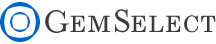X ウィンドウを閉じるX ウィンドウを閉じるX ウィンドウを閉じるサイズとウェイト Gems are always measured in Millimeter (mm) Dimensions are given as; length x width x depth, except for round stones which are; diameter x depth Select gems by size, not by weight! Gem varieties vary in density, so carat weight is not a good indication of size Note: 1ct = 0.2g